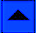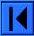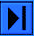The OEIS Foundation is supported by donations from users of the OEIS and by a grant from the Simons Foundation.Hints (Greetings from The On-Line Encyclopedia of Integer Sequences!)

## (Page 6)## Finding the Latest Information About a Sequence

Another use for the database is in finding out the present state of knowledge about a particular sequence.

For example, one of the classical hard problem in combinatorics is the enumeration of (labeled) partially ordered sets, or posets.

Many reference books list the first few terms, the numbers of posets with 1, 2, 3, 4, ... elements, which are:

1, 3, 19, 219, 4231, 130023, ...

To find out the latest information about this sequence, you go to the main web page of the OEIS and enter the sequence, as in the previous demonstrations.

This produces the following response.### Greetings from the On-Line Encyclopedia of Integer Sequences!

 A001035 Number of partially ordered sets ("posets") with n labeled elements (or labeled acyclic transitive digraphs). (Formerly M3068 N1244) 22
 1, 1, 3, 19, 219, 4231, 130023, 6129859, 431723379, 44511042511, 6611065248783, 1396281677105899, 414864951055853499, 171850728381587059351, 98484324257128207032183, 77567171020440688353049939, 83480529785490157813844256579, 122152541250295322862941281269151, 241939392597201176602897820148085023 (list; graph; refs; listen; history; edit; internal format)
 OFFSET 0,3 REFERENCES G. Birkhoff, Lattice Theory, Amer. Math. Soc., 1961, p. 4. J. I. Brown and S. Watson, The number of complements of a topology on n points is at least 2^n (except for some special cases), Discr. Math., 154 (1996), 27-39. K. K.-H. Butler, A Moore-Penrose inverse for Boolean relation matrices, pp. 18-28 of Combinatorial Mathematics (Proceedings 2nd Australian Conf.), Lect. Notes Math. 403, 1974. K. K.-H. Butler and G. Markowsky, Enumeration of finite topologies, Proc. 4th S-E Conf. Combin., Graph Theory, Computing, Congress. Numer. 8 (1973), 169-184. L. Comtet, Advanced Combinatorics, Reidel, 1974, pp. 60, 229. M. Erne, Struktur- und Anzahlformeln fuer Topologien auf endlichen Mengen, PhD dissertation, Westfaelische Wilhelms-Universitaet zu Muenster, 1972. M. Erne and K. Stege, Counting Finite Posets and Topologies, Order, 8 (1991), 247-265. M. Erne and K. Stege, The number of labeled orders on fifteen elements, personal communication. J. W. Evans, F. Harary and M. S. Lynn, On the computer enumeration of finite topologies, Commun. ACM, 10 (1967), 295-297, 313. J. Heitzig and J. Reinhold, The number of unlabeled orders on fourteen elements, Order 17 (2000) no. 4, 333-341. D. J. Kleitman and B. L. Rothschild, Asymptotic enumeration of partial orders on a finite set, Trans. Amer. Math. Soc., 205 (1975) 205-220. G. Kreweras, Denombrement des ordres etages, Discrete Math., 53 (1985), 147-149. A. Shafaat, On the number of topologies definable for a finite set, J. Austral. Math. Soc., 8 (1968), 194-198. N. J. A. Sloane, A Handbook of Integer Sequences, Academic Press, 1973 (includes this sequence). N. J. A. Sloane and Simon Plouffe, The Encyclopedia of Integer Sequences, Academic Press, 1995 (includes this sequence). R. P. Stanley, Enumerative Combinatorics, Cambridge, Vol. 1, Chap. 3, pages 96ff; Vol. 2, Problem 5.39, p. 88. LINKS G. Brinkmann,  B. D. McKay, Posets on up to 16 Points, Order 19 (2) (2002) 147-179 P. J. Cameron, Sequences realized by oligomorphic permutation groups, J. Integ. Seqs. Vol. 3 (2000), #00.1.5. S. R. Finch, Transitive relations, topologies and partial orders Institut f. Mathematik, Univ. Hanover, Erne/Heitzig/Reinhold papers N. Lygeros and P. Zimmermann, Computation of P(14), the number of posets with 14 elements: 1.338.193.159.771 G. Pfeiffer, Counting Transitive Relations, Journal of Integer Sequences, Vol. 7 (2004), Article 04.3.2. Bob Proctor, Chapel Hill Poset Atlas Y. Puri and T. Ward, Arithmetic and growth of periodic orbits, J. Integer Seqs., Vol. 4 (2001), #01.2.1. D. Rusin, Number of topologies N. J. A. Sloane, Classic Sequences FORMULA Related to A000798 by A000798(n) = Sum Stirling2(n, k)*A001035(k). Related to A000112 by Erne's formulae: A001035(n+1)=-s(n, 1), A001035(n+2)=n*A001035(n+1)+s(n, 2), A001035(n+3)=binomial(n+4, 2)*A001035(n+2)-s(n, 3), where s(n, k)=sum(binomial(n+k-1-m, k-1)*binomial(n+k, m)*sum((m!)/(number of automorphisms of P)*(-(number of antichains of P))^k, P an unlabeled poset with m elements), m=0..n). EXAMPLE R. P. Stanley, Enumerative Combinatorics, Cambridge, Vol. 1, Chap. 3, page 98, Fig. 3-1 shows the unlabeled posets with <= 4 points. CROSSREFS Cf. A000798 (labeled topologies), A001930 (unlabeled topologies), A000112 (unlabeled posets), A006057. Sequence in context: A005647 A158876 A001833 * A166380 A136652 A136504 Adjacent sequences:  A001032 A001033 A001034 * A001036 A001037 A001038 KEYWORD nonn,nice AUTHOR N. J. A. Sloane (njas(AT)research.att.com). EXTENSIONS Terms for n=15 and 16 from Jobst Heitzig (heitzig(AT)math.uni-hannover.de), Jul 03 2000 a(17) and a(18) from Herman Jamke (hermanjamke(AT)fastmail.fm), Mar 02 2008Click the single right arrow to go to the next demonstration page, or the single left arrow to return to the previous page.Lookup | Welcome | Wiki | Register | Music | Plot 2 | Demos | Index | Browse | More | WebCam
Contribute new seq. or comment | Format | Style Sheet | Transforms | Superseeker | Recent
The OEIS Community | Maintained by The OEIS Foundation Inc.

Last modified July 9 19:46 EDT 2020. Contains 335545 sequences. (Running on oeis4.)